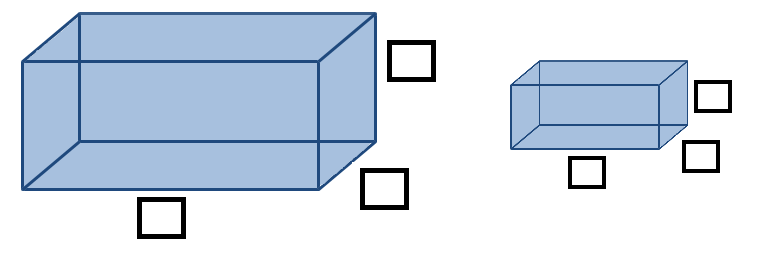Home > Grade 5 > Volume of Rectangular Prisms

# Volume of Rectangular Prisms

Directions: Using the digits 1 through 9, at most one time each, fill in the boxes to create 2 rectangular prisms so the volume of one rectangular prism is double the volume of the other rectangular prism.### Hint

How can we find the volume of a rectangular prism?
What does it mean for one quantity to be double another’s?

There are many possible answers.
One possible answer is a rectangular prism with dimensions of 2 by 8 by 9 would have a volume of 144 cubic units which is double the volume of one with dimensions of 4 by 3 by 6 (72 cubic units).

Another possible way is an 8 by 6 by 1 (48 cubic units) is double one with dimensions of 2 by 3 by 4 (24 cubic units).

Source: Joe Schwartz

## Multiplying Decimals to Make a Whole Number Product

Directions: Using the digits 1 to 9, at most one time each, fill in the …

1.Joe Schwartz,
This is a great problem!

However, I found the directions to be some what misleading. They say to use the digits 1 – 9, EACH only once. I expected to be using nine digits for the six dimensions, which means three of the dimensions would need to be double digit numbers. When I checked the answer, I saw that you did not intend for EACH DIGIT to be used.
Perhaps you intended that I was to select only single digit numbers from the digits 1-9, and that no digit could be used more than once?

Do you think there is a solution for the problem as originally written, using each of the digits 1-9 only once?

•Thanks, I updated the instructions to make this clearer.

2.Should the directions read “the volume of one rectangular prism is double the volume of the other rectangular prism”? You can’t find the volume of a rectangle…at least you couldn’t the last time I checked 🙂

•I love this problem. When I first attempted this problem I was thinking in terms of volume formula and I fell flat on my face. I realized in my second attempt that I had to actually visualize the layers within the prisms and then it was easy. What a great task for conceptual knowledge! Also, I agree with Sarah, the wording should be rectangular prism not rectangle.

•Yep. You are both right and this has been corrected.

3.4.